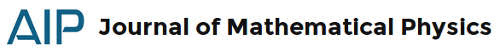### JMP 58, 1: magnetic monopoles, spacetime and gravityJust another selection of papers from the Journal of Mathematical Physics. I would start with the folowing paper:
Fine, D., & Sawin, S. (2017). Path integrals, supersymmetric quantum mechanics, and the Atiyah-Singer index theorem for twisted Dirac Journal of Mathematical Physics, 58 (1) DOI: 10.1063/1.4973368
Feynman’s time-slicing construction approximates the path integral by a product, determined by a partition of a finite time interval, of approximate propagators. This paper formulates general conditions to impose on a short-time approximation to the propagator in a general class of imaginary-time quantum mechanics on a Riemannian manifold which ensure that these products converge. The limit defines a path integral which agrees pointwise with the heat kernel for a generalized Laplacian. The result is a rigorous construction of the propagator for supersymmetric quantum mechanics, with potential, as a path integral. Further, the class of Laplacians includes the square of the twisted Dirac operator, which corresponds to an extension of $N = 1/2$ supersymmetric quantum mechanics. General results on the rate of convergence of the approximate path integrals suffice in this case to derive the local version of the Atiyah-Singer index theorem.
Kováčik, S., & Prešnajder, P. (2017). Magnetic monopoles in noncommutative quantum mechanics Journal of Mathematical Physics, 58 (1) DOI: 10.1063/1.4973503
We discuss a certain generalization of the Hilbert space of states in noncommutative quantum mechanics that, as we show, introduces magnetic monopoles into the theory. Such generalization arises very naturally in the considered model, but can be easily reproduced in ordinary quantum mechanics as well. This approach offers a different viewpoint on the Dirac quantization condition and other important relations for magnetic monopoles. We focus mostly on the kinematic structure of the theory, but investigate also a dynamical problem (with the Coulomb potential).
Laptev, A., & Maad Sasane, S. (2017). Perturbations of embedded eigenvalues for a magnetic Schrödinger operator on a cylinder Journal of Mathematical Physics, 58 (1) DOI: 10.1063/1.4974360
Perturbation problems for operators with embedded eigenvalues are generally challenging since the embedded eigenvalues cannot be separated from the rest of the spectrum. In this paper, we study a perturbation problem for embedded eigenvalues for a magnetic Schrödinger operator, when the underlying domain is a cylinder. The magnetic potential is $C^2$ with an algebraic decay rate as the unbounded variable of the cylinder tends to $\pm \infty$. In particular, no analyticity of the magnetic potential is assumed. We also assume that the embedded eigenvalue of the unperturbed problem is not the square of an integer, thus avoiding the thresholds of the continuous spectrum of the unperturbed operator. We show that the set of nearby potentials, for which a simple embedded eigenvalue persists, forms a smooth manifold of finite codimension.
Krongos, D., & Torre, C. (2017). Rainich conditions in (2 + 1)-dimensional gravity Journal of Mathematical Physics, 58 (1) DOI: 10.1063/1.4974091
In (3 + 1) spacetime dimensions, the Rainich conditions are a set of equations expressed solely in terms of the metric tensor which are equivalent to the Einstein-Maxwell equations for non-null electromagnetic fields. Here we provide the analogous conditions for (2 + 1)-dimensional gravity coupled to electromagnetism. Both the non-null and null cases are treated. The construction of these conditions is based upon reducing the problem to that of gravity coupled to a scalar field, which we have treated elsewhere. These conditions can be easily extended to other theories of (2 + 1)-dimensional gravity. For example, we apply the geometrization conditions to topologically massive gravity coupled to the electromagnetic field and obtain a family of plane-fronted wave solutions.
Imaykin, V., Komech, A., & Spohn, H. (2017). On invariants for the Poincaré equations and applications Journal of Mathematical Physics, 58 (1) DOI: 10.1063/1.4973552
A special version of the Noether theory of invariants is developed for the Poincaré equations. This theory is applied to the Maxwell equations coupled to the Abraham rotating extended electron with the configuration space SO(3).
See you for the next digest!